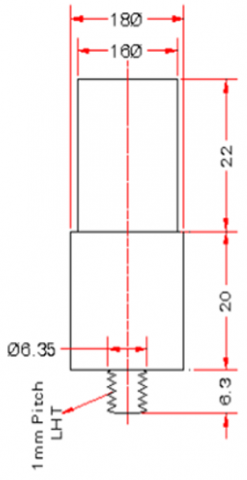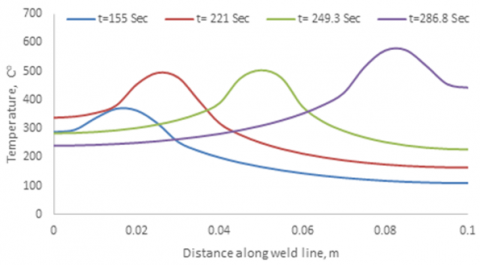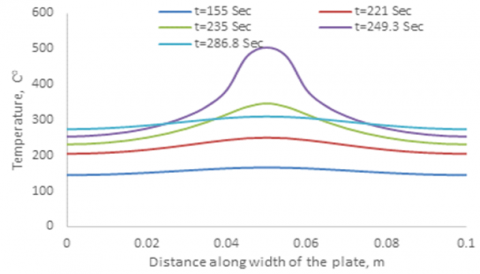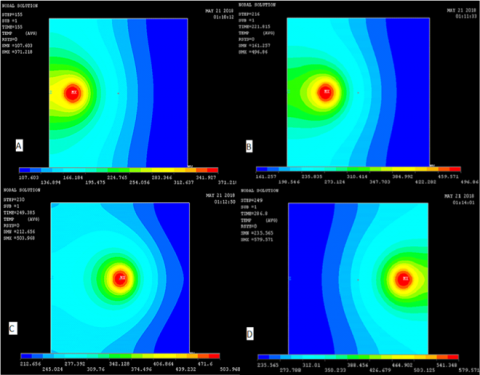# Numerical validation of temperature distribution in friction stir welded aluminum 7075-T651 plates using pseudo heat transfer model

Numerical validation of temperature distribution in friction stir welded aluminum 7075-T651 plates using pseudo heat transfer model

Kareem N Salloomi  Sanaa Al-Sumaidae

Automated Manufacturing Department Al-Khwarizmi College of Engineering, University of Baghdad, Baghdad, 10071, Iraq

Corresponding Author Email:
Page:
29-38
|
DOI:
https://doi.org/10.3166/ACSM.41.29-38
|
Accepted:
|
Published:
30 June 2017
| Citation

OPEN ACCESS

Abstract:

The current paper develops a three-dimensional nonlinear finite element model to investigate temperature distribution during friction stir welding (FSW) of Aluminum 7075-T651 plates. To achieve the purpose, an ANSYS APDL code was built to construct the three-dimensional plates geometry, assigning nonlinear material properties, discretize model mesh, and later doing the transient thermal analysis of temperature distribution using moving pseudo heat model. The local heat generated due to friction between tool shoulder and tool pin with the workpiece was applied as a moving heat input (Q). The FSW model accounts for all process stages such as: plunging, dwelling, traversing, pulling out and finally cooling of the work piece.  To simulate the real situation of heat transfer during FSW as closely as possible, the effects of different heat transfer conditions such as heat losses due to convection and radiation surrounding workpiece and thermal contact conductance between work-piece and the backing plate interface on the thermal history of the weld material were considered. The results obtained throughout the current simulation were in a good agreement with a previous published experimental result

Keywords:

friction stir welding (FSW), finite element simulation, AL 7075-T651, thermal modeling

1. Introduction

The 7xxx series aluminum alloys have properties that are convenient for high-strength aerospace and automotive structural engineering applications. The alloy series can be used as aircraft fittings, gears and shafts, fuse parts, meter shafts, missile parts, regulating valve parts, bike frames, and all-terrain vehicle (ATV) sprockets etc. The reason behind this wide usage is that the 7xxx series offer advanced and lightweight properties like good strength-to-weight ratio, corrosion resistance and machinability. Among these alloys is AL-7075-T651 which is promising due to both: its high strength to weight ratio and high response to natural age hardening. The high strength properties are basically attained through the precipitation of Mg2Zn and Al2CuMg phases. Despite the above achieved mechanical properties, welding of high strength alloys like the 7xxx series pose remarkable challenges. The fusion welding of alloy such as AL-7075 using conventional methods is usually difficult to be done without serious problems due to weld solidification cracking. With the introduction of friction stir welding process (FSW) some of solidification-related welding defects were eliminated such as cracks, porosity, evaporative loss, etc.. The process classified as a new joining technology that keeps up to 90% of the mechanical properties of aluminum alloys (Mishra and Komarasamy, 2016; Taendl et al., 2013; Kim et al., 2016). As a process, FSW depends both on heat and material flow, which in turn involves occurring of plastic deformation to initiate softening and displacement of a finite volume of workpiece material, i.e. material in the direct vicinity of the joining tool (Guerra et al., 2003). For this reason, FSW has been characterized as a thermo-mechanical process that involves hot working deformation.

Due to its importance as a structural component, many experimental research studies have already been conducted on 7075 aluminum alloys to optimize input FSW parameters (tool speed, feed rate, pin shape, tilt angle and tool depth). The effect of using different rotational and traverse speeds on microstructural changes, mechanical properties and residual stress distribution of 7075-T651 Al alloy had been investigated by several studies (Shah and Badheka, 2016; Çevik et al., 2016; Rao et al., 2015). Higher tensile strength was achieved at medium rotational and welding speeds. The inspection tests showed that, the friction stir welded plates had suffered from tunnel defects in the root of the weld line due to both improper plunge depth and inappropriate stirring of the material around the tool.  Moreover, microvoids and large grain bands were formed on the retreating side of the weld line at high speed causing insufficient filling in the joints. In fact, the knowledge of temperature distribution in the stirred volume and around the processed zone is effective as it controls the microstructural evolution. Several experimental studies were carried out to measure the temperature in the region just outside the welded line as it is relatively simple and avoids the complexity arising due to the high deformation in the stirred zone (Mahoney et al., 1998; Colegrove and Shercliff, 2003; Upadhyay and Reynolds, 2010). The aforementioned literature used embedded thermocouples as tools to measure the peak temperature as well as temperature history during the friction stir welding of the samples at some specific locations away from the nugget zone. However, the process of measuring temperature variations in the nugget zone using the thermocouples is a very complex task. To overcome this shortcoming, numerical methods such as finite element method were adopted as an efficient and convenient procedures to analyze and visualize material flow, temperature field, stresses and strains involved during the FSW process. Therefore, in order to obtain the optimal weld properties, simulations can help to adjust and optimize the process parameters and tool design (Yaduwanshi et al., 2015; Ramesh et al., 2015; Buffa et al., 2006). In previous simulations studies of FSW, two types of process modeling techniques were implemented: the first is the fluid dynamics procedure which involves simulation of material flow and temperature distribution while the second is the solid mechanics and that involves simulation of temperature distribution and subsequent stresses and strains. The two modeling techniques adopt non-linear phenomena that belongs to the consideration of three classical types: geometric, material or contact nonlinearity.

It is worthy to mention that most of the work done with the aspect of friction stir welding of 7xxx series aluminum alloys used the experimental approach to predict the thermal history or mechanical strength of the resulted joint. To date, most of the numerical researches literature have been done on the heat transfer of FSW of 6xxx & 2xxx series aluminum alloys due to nonlinear data availability while a big shortage in the analysis of 7xxx series is exist. So, the objective of this paper is to simulate the friction stir welding of AL-7075-T651 by constructing a solid mechanics three-dimensional model that accounts for the geometrical and material nonlinearity. The numerical results were validated by comparing with the thermal history obtained earlier by a previous experimental study carried out by reference (Shah and Badheka, 2016).

2. Finite element and heat transfer model

In order to capture the transient temperature profile during the friction stir welding of AL-7075-T651, a three-dimensional nonlinear finite element model was constructed using ANSYS 14 simulator. The model considers both geometrical and material nonlinearity. The experimental data of the current model were taken from reference (Shah and Badheka, 2016) in which two plates of dimensions 100mm x 50mm of AL-7075-T651 were joined together using friction stir welding process. The weld was performed at 50 mm/min feed speed and 1500 rpm rotational speed. The design of the tool used in the welding process is shown in Figure 1.

To perform the thermal analysis, an algorithm was built using the ANSYS APDL Macro language that accounts for all process stages such as: plunging, dwelling, traversing, pulling out and finally cooling of the work piece. For meshing purpose, the Pseudo heat transfer model used the 3-D solid element SOLID70, which has eight nodes each with a single temperature degree of freedom. To account for the convection and radiation effect on the plate surface, a three-dimensional thermal surface effect element (SURF152) is used overlaying it onto the faces of the base elements made by SOLID70. The final model was meshed with a total of 19220 elements.

## 1.pngFigure 1. Dimensions of the tool

The governing equation for the transient temperature distribution in workpiece or thermal analysis of welding is like the governing equation of 3-D heat conduction with suitable boundary condition:

$\nabla\left(K_{k} \nabla T\right)+Q=\rho c \partial T / \partial t$    (1)

Where, ρ is material density kg/m3, C specific heat in J/kg.Co,  Kk thermal conductivity along x, y, and z directions in W/m.Co, T Absolute temperature in Co and Q is local heat generated by friction. In this simulation, the local heat Q generated by friction between the tool and surface of welding workpiece is modeled using derived in reference (Riahi and Nazari, 2011) and is given by:

$Q=\frac{4 \pi^{2}}{3} \mu N P\left(r_{S}^{3}+3 r_{p}^{2} h\right)$   (2)

Where, µ is the friction coefficient between tool and plates and it is assumed 0.3. N is the rotational speed, P is the pressure applied by the tool and assumed to be uniform on tool/work piece interface surfaces, rs is shoulder radius, rp is pin radius, and h is plate thickness.

To account for the tool translation along weld length, a Pseudo moving heat source was considered in the transient modeling. The traveling speed of the tool (Vt) was simulated by changing heat source location according to the following criteria (Salloomi et al., 2013):

$X_{i+1}=X_{i}+V_{t} \Delta t$       (3)

Where, ∆t is the time required for the tool to travel from Xi location to Xi+1 (i.e element size) and Vt is the tool traveling speed which is considered as a constant equal to the feed speed of FSW. Figure 2 illustrates concept of applying moving heat source in simulation of friction stir welding.

## 2.pngFigure 2. Concept modeling of friction stir welding moving heat source

To simplify the numerical solution of Eq.1, the heat transfer boundary conditions that surrounding the FSW process were applied. Heat losses from the workpiece surfaces to the surroundings were considered due to both natural convection and radiation effects.

For the radiant heat loss, qr

$q_{r}=\sigma \epsilon_{r}\left(T_{r}^{4}-T_{\infty}^{4}\right)$   (4)

Where, s=the Stefan-Boltzmann constant (σ=5.67*10-8 W/m2.Co), er=the emissivity of a radiating surface (er= 0.5), Tr = the absolute temperature of radiating surface, T = the absolute temperature of surrounding (T=25 Co).

For the convective heat loss, qc

$q_{c}=h_{f}\left(T_{s}-T_{\infty}\right)$    (5)

Where, hf= the convection coefficient (hf=30 W/m2.Co) , Ts = the temperature at the plate surfaces.

The conduction heat transfer between the bottom surface of the workpiece and the backing supporting plate is considered by using a larger convective coefficient (hf=300 W/m2.Co). This was assumed due to the high difficulty in obtaining an accurate value for the gap conductivity between workpiece and backing supporting plate.

The material nonlinearity was considered in this work through using material thermal properties that are varying with temperature (Mills, 2002). Table 1. shows variation of material density, thermal conductivity, and specific heat capacity versus temperature change for 7075 Aluminum alloys.

Table 1. Thermo-physical propertites of 7075 aluminum alloy

 Temperature Co Density kg/m3 Specific Heat J/kg.Co Conductivity W/m.Co 25 2805 850 130 100 2795 910 186 200 2770 960 197 300 2750 980 194 400 2725 1040 196 500 2700 1100 196 532 2692 1110 193
3. Results and discussion

To validate numerical results of the current proposed finite element model, a comparison of the temperature history was made with the experimental results obtained by reference (Shah and Badheka, 2016). In the work done by (Shah and Badheka, 2016), two thermocouples were placed at a distance of 15mm from the center weld line to measure the temperature distribution; one on the advancing side and other on the retreating side of the weld joint. For that purpose, two holes with a diameter of 2mm and depth of 35mm were drilled on both sides at the center in the thickness direction to accommodate the thermocouples.

## 3.pngFigure 3. Comparison of the numerical and measured thermal history of a point at a distance of 15 mm from the weld line

Figure 3 shows the temperature history obtained through using the proposed finite element model for the same point indicated above by the thermocouple location. The figure shows good correlation between numerical and experimental results except at the range of temperature above 400 Co which might happen due to the sudden removal of the heat source in the numerical simulation. A 2.3% error between the numerical & advanced side experimental value of the peak temperature was recorded; this indicate high efficiency of applying numerical simulation as a tool to predict thermal behavior of friction stir welded material. A large discrepancy occurs after 400 Sec, with the cooling rate higher in the FE model. This is happening due to the assumed constant convection constant used in the numerical model.

## 4.pngFigure 4. Temperature distribution for four different tool positions along weld line

Figure 4 shows temperature distribution for four different tool positions along weld line. The figure shows clearly that the peak temperature through the FSW process is increasing with time and that happen due to energy tapping in the welded specimen. The figure also indicates the effect of thermal conductivity variation on temperature distribution; it simply made the distribution wider for higher temperatures than the lower ones.

## 5.pngFigure 5. Temperature distribution for a weld path positioned at the mid-length of the plate

Figure 5 shows the temperature distribution for a weld path positioned at mid-length of the plate across the width. The results indicate temperature increasing of the welded workpiece as the tool approaches the weld path position and then it is clearly shown at time 286.8 Sec the temperature will decrease since the tool passed the weld path and reached the other end of the plate. To verify the ability of the computational model to effectively measure temperature changes in some critical zones like nugget zone, the transient temperature distribution through plate thickness for the weld path positioned at plate mid-length is plotted in Figure 6. Figure 7 A, B, C, and D shows contours of temperature variation on plate surface as the tool moves along weld line. It is clear that the temperature increases as the tool moves away from the start point due to preheating effect.

## 6.pngFigure 6. Temperature contours through plate thickness for a weld path positioned at the mid-length of the plate ; A at 155 Sec, B at 221 Sec, C at 249.3 Sec, D at 286.8 Sec

## 7.pngFigure 7. Temperature contours during FSW of AL 7075-T651 using moving heat source; A at 155 Sec, B at 221 Sec, C at 249.3 Sec, D at 286.8 Sec

4. Conclusions

A three-dimensional finite element model was constructed to validate the experimental temperature distribution of a former study for the FSW of 7075-T651 aluminum alloy. A good match between the computed and experimental curves was deducted. The finite element results show that the high gradient in the temperature is in the region under the shoulder. Moreover, the computational analysis used in this work showed the ability of predicting the temperature in critical zones like nugget zone which is extremely hard to be measured using experimental methods.

References

Buffa G., Hua J., Shivpuri R., Fratini L. (2006). Design of the friction stir welding tool using the continuum-based FEM model. Materials Science and Engineering A, Vol. 419, No. 1-2, pp. 381-388. https://doi.org/10.1016/j.msea.2005.09.041

Çevik B., Özçatalbaş Y., Gülenç B. (2016). Friction stir welding of 7075-T651 aluminum alloy. Practical Metallography, Vol. 53, No. 1, pp. 6-23.

Colegrove P. A., Shercliff H. R. (2003). Experimental and numerical analysis of aluminum alloy 7075-T7351 friction stir welds. Science and Technology of Welding and Joining, Vol. 8, No. 5, pp. 360-368. https://doi.org/10.1179/136217103225005534

Guerra M., Schmidt C., McClure J. C., Murr L. E., Nunes A. C. (2003). Flow patterns during friction stir welding. Materials Characterisation, Vol. 49, No. 2, pp. 95-101. http://dx.doi.org/10.1016/S1044-5803(02)00362-5

Kim Y. G., Kim I. J., Kim Y. P., Joo S. M. (2016). A feasibility study on the three-dimensional friction stir welding of aluminum 5083-O thin plate. Materials Transactions, Vol. 57, No. 6, pp. 988-994. http://dx.doi.org/10.2320/matertrans.M2015475

Mahoney M. W., Rhodes C. G., Flintoff J. G., Spurling R. A., Bingel W. H. (1998). Properties of friction-stir-welded 7075 T651 aluminum. Metallurgical and Materials Transactions A, Vol. 29, No. 7, pp. 1955-1964. https://doi.org/10.1007/s11661-998-0021-5

Mills K. C. (2002). Recommended Values of Thermophysical Properties for Selected Commercial Alloys. National Physical Laboratory and ASM International, Woodhead Publishing Limited, Cambridge, England, pp. 68-71.

Mishra R. S., Komarasamy M. (2016). Friction Stir Welding of High-Strength 7XXX Aluminum Alloys. Butterworth-Heinemann, UK, p. 104.

P. H., Badheka V. (2016). An experimental investigation of temperature distribution and joint properties of Al 7075 T651 friction stir welded aluminum alloys. Procedia Technology, Vol. 23, pp. 543-550. http://dx.doi.org/10.1016/j.protcy.2016.03.061

Ramesh R., Kumar S. S., Sivaraman V., Mohan R. (2015). Finite element analysis and simulation of Al 7075 alloy joints produced by friction stir welding. Applied Mechanics and Materials, Vol. 766-767, pp. 1116-1120. https://doi.org/10.4028/www.scientific.net/AMM.766-767.1116

Rao T. S., Reddy G. M., Rao S. R. K. (2015). Microstructure and mechanical properties of friction stir welded AA7075–T651 aluminum alloy thick plates. Transactions of Nonferrous Metals Society of China, Vol. 25, No. 6, pp. 1770-1778. http://dx.doi.org/10.1016/S1003-6326(15)63782-7

Riahi M., Nazari H. (2011). Analysis of transient temperature and residual thermal stresses in friction stir welding of aluminum alloy 6061-T6 via numerical simulation. The International Journal of Advanced Manufacturing Technology, Vol. 55, No. 1-4, pp. 143-152. https://doi.org/10.1007/s00170-010-3038-z

Salloomi K. N., Sabri L. A., Hamad Y. M., Mohammed S. N. (2013). 3-Dimensional nonlinear finite element analysis of both thermal and mechanical response of friction stir welded 2024-T3 aluminum plates. Journal of Information Engineering and Applications, Vol. 3, No. 9, pp. 6-15.

Taendl J., Nambu S., Inoue J., Enzinger N., Koseki T. (2013). Friction stir welding of multilayered steel. Science and Technology of Welding and Joining, Vol. 17, No. 3, pp. 244-253. https://doi.org/10.1179/1362171812Y.0000000003

Upadhyay P., Reynolds A. P. (2010). Effects of thermal boundary conditions in friction stir welded AA7050-T7 sheets. Materials Science and Engineering A, Vol. 527, No. 6, pp. 1537-1543. https://doi.org/10.1016/j.msea.2009.10.039

Yaduwanshi D. K., Bag S., Pal S. (2015). Heat transfer analyses in friction stir welding of aluminium alloy. Proc IMechE Part B: J Engineering Manufacture, Vol. 229, No. 10, pp. 1722-1733